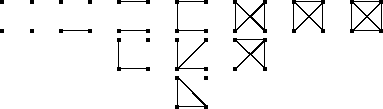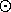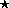Cycle indicator polynomials

## Cycle indicator polynomials

We have seen in Pólya's Theorem that the generating function for the enumeration of the G-classes on YX by weight is equal to
(1)/( | G | )ågÎG Õi=1 | X | ( åyÎYyi)ai(bar (g)).
This polynomial can be obtained from the polynomial
C(G,X):=(1)/( | G | )ågÎGÕi=1 | X | ziai(bar (g))ÎQ[z1,...,z | X | ]
by simply replacing the indeterminate zi by the polynomial åyyi. It is therefore the polynomial C(G,X) which really matters. We call this polynomial the cycle indicator polynomial or the cycle index of GX, since it displays the cycle structure of the elements bar (g)Îbar (G). (But it should be mentioned that C(G,X)=C(H,X) does not mean that bar (G) and bar (H) are isomorphic. There are counterexamples known: for example, the regular representation of the nonabelian group of order p3, p an odd prime number, has the same cycle indicator polynomial as the regular representation of the abelian group Cp´Cp´Cp (exercise.) In the case when we wish to display its indeterminates we shall write C(G,X;z1,...,z | X | ), and if we replace zi by riÎQ, we simply write C(G,X;r1,...,r | X | ). Hence we obtain, for example (cf. Theorem):
Corollary: The number of G-classes on YX is equal to
C(G,X; | Y | ,..., | Y | ).
In order to generalize the substitution process mentioned above which yields the generating function from C(G,X), we put, for any polynomial p in Q[u1,...,um],
C(G,X | p(u1,...,um))=(1)/( | G | )ågÎG Õi=1 | X | p(u1i,...,umi)ai(bar (g))ÎQ[u1, ...],
and call this process Pólya-substitution. Using this notation we can rephrase corollary as follows:
Pólya's Theorem The generating function for the enumeration of G-classes on YX by content can be obtained from the cycle indicator of GX by Pólya-substituting åyÎYy into the cycle indicator polynomial C(G,X). Hence this generating function is equal to
C(G,X | åyÎYy).
In order to count G-classes by content it therefore remains to evaluate the cycle indicator of GX. A few examples should be welcome:
Examples:  of cycle indicator polynomials
• The cycle indicator of the identity subgroup of Sn and its natural action on n is
C({1},n)=z1n.
• The cycle indicator of the natural action of the cyclic group Cn on n is (recall the corollary on the number of different necklaces)
C(Cn,n)=(1)/(n)åd | nf(d)zdn/d.
• The cycle indicator of the natural action of the dihedral group Dn of order 2n on the set n (which can be considered as being the set of vertices of the regular n-gon, of which Dn is the symmetry group) satisfies
C(Dn,n)=(1)/(2)C(Cn,n)+(1)/(2)z1z2(n-1)/2 if n is odd,
C(Dn,n)=(1)/(2)C(Cn,n)+(1)/(4)(z2n/2+z12z2(n-2)/2) if n is even.
This follows from the fact that the rotations form a cyclic subgroup of order n, while the remaining reflections either leave exactly one vertex fixed (in the case when n is odd) or two vertices or none (in the case when n is even), and group the remaining vertices into pairs of vertices that are mapped onto each other.
• Furthermore we have the following cycle indicators of the natural actions of Sn and An (cf. the descripton of the conjugacy classes of Sn and exercise):
C(Sn,n)=åa|¾| nÕk(1)/(ak!) ((zk)/(k))ak,
C(An,n) = åa|¾| n(1+(-1)a2+a4+...) Õk(1)/(ak!)((zk)/(k))ak.

For the graphs on 4 vertices we use the cycle index (exercise)

C(S4,[4 choose 2])= (1)/(24)(z16+9z12z22+8z32+ 6z2z4),
which is obtained from the corollary on the induced action on the set of 2-sets. It yields the generating function
C(S4,[4 choose 2] | y0+y1)= y06+y05y1+2y04y12+ 3y03y13+2y02y14+y0y15+y16,
in accordance with figure.The graphs on 4 points

In order to make life easier we can replace y0+...+ym-1 by 1+y1+...+ym-1, and in the case when | Y | =2 we can even take 1+y instead of y0+y1 or 1+y1,so that, for example, the generating function for the graphs on 4 vertices by their number of edges takes the following form:

C(S4,[4 choose 2] | 1+y)= 1+y+2y2+3y3+2y4+y5+y6.
Here the coefficient of ye is equal to the number of graphs on 4 vertices which possess exactly e edges, so that we obtain (recall the corollary on the number of k-graphs on v vertices and the corollary on selfcomplementary graphs):
Corollary: The total number of graphs on v vertices is equal to
C(Sv,[v choose 2];2,...,2),
while the number of selfcomplementary graphs on v vertices is equal to
C(Sv,[v choose 2];0,2,0,2,...).
The number of graphs on v vertices which contain e edges is the coefficient of ye in the polynomial
C(Sv,[v choose 2] | 1+y).
These examples have shown that the evaluation of the cycle indicator polynomial is an important step towards the solution of various enumeration problems, so that a few remarks concerning this are well in order. The cycle indicators of the natural actions of Sn , An , Cn , and Dn are at hand and we therefore put the question how we can obtain cycle indicators of groups, which are direct sums or certain products of symmetric, alternating, cyclic or dihedral groups. More generally we want to know how the cycle indicator of a sum or a product can be obtained from the cycle indicators of the summands or factors, depending of course on the action in question. The following first remarks are clear from the definitions of direct sum and cartesian product:
Lemma: Let GX and HY denote finite actions. We have
C(G´H,X DOTCUP Y)=C(G,X)·C(H,Y),
and
C(G´H,X´Y)=(1)/( | G | | H | ) å(g,h) Õi,k=1 | X | | Y | zlcm(i,k)gcd(i,k)ai(bar (g) )ak(bar (h)).
Further important constructions are the plethysm HG, and the composition. They are defined as permutation groups induced by similar actions of H wr X G , and so the corresponding cycle indicator polynomials are the same:
C(G[H],Y´X)=C(HG, | Y | | X | ).
From the description of the conjugacy classes of elements of complete monomial groups we obtain for C(HG, | Y | | X | ) the following plethysm of cycle indicators :
C(H,Y)C(G,X):=(1)/( | G | )åg Õk=1 | X | ((1)/( | H | )åhÕi=1 | Y | zi·kai(bar (h)))ak(bar (g)).
Finally we show how the cycle indicator polynomial C(G,X) can be obtained from the character c:g -> | Xg | of the action GX in question. In order to do this we have to evaluate the numbers ai(bar (g)),iÎN, for each gÎG, and we shall do this by inverting the equations
c(gk)=a1(bar (gk))=a1(bar (g)k)=åd | kd·ad(bar (g))
(cf. the lemma describing the powers of a cycle for the last equation). The inversion procedure uses the number theoretic Moebius function and the corresponding inversion theorem, but as we are going to use also other Moebius functions later on we shall describe this inversion method in a slightly more general context than is actually needed here.

We start doing so by introducing the notion of incidence algebra. Let (P, <= ) denote a poset (partially ordered set). Its partial order <= allows us to introduce intervals  [p,q]:={rÎP | p <= r <= q}. If all these intervals are finite, then (P, <= ) is called a locally finite poset. Assuming this and denoting by F a field, we can turn the set

IF(P):={j:P2 -> F | j(p,q)=0 unless p <= q}
of all the incidence functions into an F-algebra. The following addition and scalar multiplication define a vector space structure:
(j+y)(p,q):=j(p,q)+y(p,q) , (rj)(p,q):= r·j(p,q), rÎF,
while the local finiteness allows to define the convolution product by
(jy)(p,q):=årÎ[p,q]j(p,r)y(r,q).
This makes IF(P) into a ring (exercise). The identity element is the Kronecker d-function
d(p,q):=1 if p=q
d(p,q):=0 otherwise.
As scalar mutiplication and convolution satisfy
r(jy)=(rj)y=j(ry),
this ring is even an F-algebra, the incidence algebra over F of (P, <= ). It is important to characterize its invertible elements, i.e. the j in IF(P) for which there exists a yÎIF(P) such that yj=d and also jy=d. We obtain these incidence functions by an easy argument from linear algebra that allows to identify the incidence functions with upper triangular matrices as follows. In the case when P is finite, we can embed the partial order into a total order (i.e. we can number the pÎP in a way such that pi<pk implies i<k). This yields a bijection between IF(P) and the set of upper triangular matrices over F which respects addition and scalar multiplication. The convolution product corresponds to the matrix product of the associated matrices
j -> F:=(j(pi,pk)),
and so j is invertible if and only if the values j(p,p) are nonzero. But this is true also in the more general case when we only assume (P,£) to be locally finite, since then we can easily show that the incidence function recursively defined in the second item of the following lemma is in fact an inverse with respect to the convolution product:
Lemma: For each locally finite partial order (P,£) and any jÎIF(P) the following is true:
• j is invertible if and only if, for each pÎP, we have
j(p,p) not = 0.
• If j is invertible, then j-1 satisfies j-1(p,p)=j(p,p)-1, and
j-1(p,q) =-j(q,q)-1årÎ[p,q)j-1(p,r)j(r,q)
=-j(p,p)-1 årÎ(p,q]j(p,r)j-1(r,q),
where as usual [p,q) and (p,q] denote half open intervals.
A specific invertible incidence function is the zeta function which describes the partial order in question:
z(p,q):=1 if p <= q,
z(p,q):=0 otherwise.
Its inverse is called the Moebius function of (P, <= ): m:=z-1, for which we obtain from the preceeding lemma the following recursions:
m(p,q)=-årÎ[p,q)m(p,r)=-årÎ(p,q]m(r,q).
This close connection between the zeta and the Moebius function provides a very useful inversion theorem which we introduce next. In a poset (P, <= ) the sets {qÎP | q <= p} are called the principal (order-)ideals of P, while the sets {qÎP | q >= p} are called the principal filters . The inversion theorem now reads as follows:
The Moebius Inversion Let (P, <= ) denote a locally finite poset and let F and G denote mappings from P into the field F. Then
• if all the principal ideals of P are finite, we have the following equivalence of systems of equations:
" p: G(p)=åq <= pF(q) iff " p: F(p)=åq <= pG(q)m(q,p).
• If all the principal filters of P are finite, we have the following equivalence of systems of equations:
" p: G(p)=åq >= pF(q) iff " p: F(p)=åq >= pm(p,q)G(q).
Proof: We prove the first statement, the second one follows analogously. We add to P an element 0 such that 0<p, for each pÎP, obtaining a new poset (bar (P)=PÈ{0}, <= ). As all the principal ideals of (P, <= ) are supposed to be finite, (bar (P), <= ) is a locally finite poset. Now we associate to F and G the incidence functions jyÎIF(bar (P)) defined by
j(p,q):=F(q) if p=0 and q>0,
j(p,q):=0 otherwise,
y(p,q):=G(q) if p=0 and q>0,
y(p,q):=0 otherwise.
The system of equations G(p)=åq <= pF(q) is equivalent to the identity y=jz, which again is equivalent to ym=j, and this is nothing but the system of equations F(p)=åq <= p G(q)m(q,p).

A particular locally finite poset is (N*, | ), the set of positive natural numbers together with divisibility as its partial order. The corresponding m is called the number theoretic Moebius function. Instead of m(p,q) one can write m(q/p) in this case since the intervals [p,q] and [r,s] are order isomorphic if q/p=s/r, and so m(p,q)=m(r,s), by the above mentioned recursion. Thus we obtain, by an application of the Moebius Inversion formula and the formula for the character of gk:

Corollary: For each finite action GX the following equivalent systems of equations hold:
" k: a1(bar (g)k)=åd | kd·ad(bar (g)) and " k: ak(bar (g))=(1)/(k) åd | km(k/d)a1(bar (g)d),
where m denotes the number theoretic Moebius function. In particular we obtain the following expression of the cycle indicator of GX in terms of the character c of GX:
C(G,X)=(1)/( | G | )ågÕizii-1Sd | i m(i/d)c(gd).
In order to apply this result, we need to know the values of the number theoretic Moebius function. As z is defined by the partial order, the same holds for the Moebius function, and hence the Moebius function is the same for order isomorphic posets. Thus the values of the Moebius function on (N*, | ) can be evaluated by noting that the Moebius function is multiplicative and that an interval [d,n], where d divides n, is order isomorphic to the cartesian product
[1,p1k1]´...´[1,prkr],
if n/d has the prime number decomposition n/d=p1k1·...·prkr, where the pi are different prime numbers and the ki >= 1. Thus, for the number theoretic Moebius function we have (exercise):
m(n/d):=m(d,n)=m(1,n/d)=Õi=1rm(1,piki).
But from the recursion for the Moebius function we can deduce that m(1)=1,m(p)=-1, if p is prime, and m(pr)=0, if r>1. This together with the description of the interval [d,n] finally yields:
m(n)=1 if n=1
m(n)=(-1)r if n is a product of r different primes,
m(n)=0 otherwise.
Examples:
• The exterior cycle index of bar (G) <= SX is defined to be the cycle indicator of the natural action of SX on the set of left cosets SX/bar (G). The permutation character of this action is (exercise)
c(p)=( | X | ! | CS(p)Çbar (G) | )/( | bar (G) | | CS(p) | ).
This together with an application of the formula for computing the cycle type yields the exterior cycle index
C(SX,SX/bar (G))
of bar (G).
• The character of SX on the set [X choose k] of all the k-subsets of X is
c(p)=åb|¾| kÕi=1k[ai(p) choose bi] ,
since a k-subset is fixed under p if and only if it is the union of cyclically permuted points. Hence we can apply the formula for computing the cycle type in order to evaluate C(G,[X choose k])= C(bar (G),[X choose k]). A particular case is C(Sv,i [v choose 2]) which we need for the enumeration of graphs on v vertices, and which is obtained from the corollary on the induced action on the set of 2-sets:
c(bar (p))=[a1(p) choose 2]+a2(p).
• Exercises

• harald.fripertinger@kfunigraz.ac.at,
last changed: August 28, 2001Cycle indicator polynomials